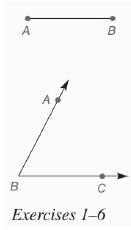Chapter 7.CR, Problem 4CRElementary Geometry For College St...

7th Edition
Alexander + 2 others
ISBN: 9781337614085

Solutions

Chapter
SectionElementary Geometry For College St...

7th Edition
Alexander + 2 others
ISBN: 9781337614085
Textbook Problem

In Review Exercises 1 to 6, use the figure shown.Construct an isosceles triangle with vertex angle B and an altitude with the length of A B ¯ from vertex B to the base.To determine

To construct: An isosceles triangle with vertex angle B and an altitude with the length of AB¯

from vertex B to the base.

Explanation

Given:

Construct an isosceles triangle with vertex angle B and an altitude with the length of AB¯

from vertex B to the base.

Calculation:

Consider the altitute of the isosceles triangle as AB¯

Still sussing out bartleby?

Check out a sample textbook solution.

See a sample solution

The Solution to Your Study Problems

Bartleby provides explanations to thousands of textbook problems written by our experts, many with advanced degrees!

Get Started

Find more solutions based on key concepts